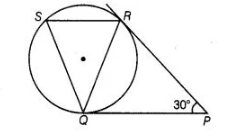# In figure, tangents PQ and PR are drawn to a circle

Question:

In figure, tangents PQ and PR are drawn to a circle such that ∠RPQ = 30°. A chord RS is drawn parallel to the tangent PQ. Find the ∠RQS.

Solution:

PQ and PR are two tangents drawn from an external point P.$\therefore \quad P Q=P R$

[the lengths of tangents drawn from an external point to a circle are equal]

$\Rightarrow \quad \angle P Q R=\angle Q R P$

[angles opposite to equal sides are equal]

Now, in $\triangle P Q R \quad \angle P Q R+\angle Q R P+\angle R P Q=180^{\circ}$

[sum of all interior angles of any triangle is $180^{\circ}$ ]

$\Rightarrow \quad \angle P Q R+\angle P Q R+30^{\circ}=180^{\circ}$

$\Rightarrow \quad 2 \angle P Q R=180^{\circ}-30^{\circ}$

$\Rightarrow \quad \angle P Q R=\frac{180^{\circ}-30^{\circ}}{2}=75^{\circ}$

Since, $S R \| Q P$

$\therefore \quad \angle S R Q=\angle R Q P=75^{\circ} \quad$ [alternate interior angles]

$\begin{array}{lll}\text { Also, } & \angle P Q R=\angle Q S R=75^{\circ} & \text { [by alternate segment theorem] }\end{array}$

$\ln \triangle Q R S$, $\angle Q+\angle R+\angle S=180^{\circ}$

[sum of all interior angles of any triangle is $180^{\circ}$ ]

$\Rightarrow \quad \angle Q=180^{\circ}-\left(75^{\circ}+75^{\circ}\right)$

$=30^{\circ}$

$\therefore \quad \angle R Q S=30^{\circ}$